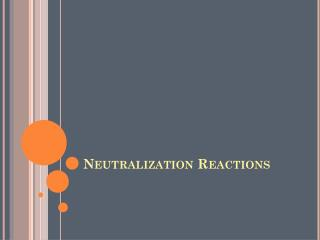DownloadDownload PresentationNeutralization Reactions

# Neutralization Reactions

Télécharger la présentation## Neutralization Reactions

- - - - - - - - - - - - - - - - - - - - - - - - - - - E N D - - - - - - - - - - - - - - - - - - - - - - - - - - -
##### Presentation Transcript

1. Neutralization Reactions

2. Neutralization Reactions • Adding a base to an acid neutralizes the acid’s acidic properties. • This type of reaction is called a neutralization reaction. • There are many different acids and bases and being able to predict the results of reactions between them is important.

3. Neutralization Reactions • Bakers depend on neutralization reactions to create light, fluffy baked goods. • Gardeners and farmers depend on these reactions to modify the characteristics of the soil. • Industrial chemists rely on these reactions to produce the raw materials that are used to make a wide variety of chemicals and chemical products.

4. Neutralization Reactions • The quantitative reaction between an acid and a base produces an ionic compound (a salt) and water. Acid + Base  A Salt + Water • A salt is any ionic compound that is composed of the anion from an acid and a cation from a base. Cation from base HNO3(aq) + NaOH(aq) NaNO3 + H2O(l) Anion from acid

5. Neutralization Reactions • The balanced chemical equation for that reaction showed that 1 mol of nitric acid reacted with 1 mol of sodium hydroxide. • If equal molar quantities of nitric acid and sodium hydroxide are used, the result is a neutral (pH 7) aqueous solution of sodium nitrate.

6. Neutralization Reactions • In fact, when any strong acid reacts with any strong base in the mole ratio from a balanced chemical equation, a neutral aqueous solution of a salt is formed. • Reactions between acids and bases of different strengths usually do not result in neutral solutions.

7. Neutralization Reactions • For most neutralization reactions, there are no visible signs that a reaction is occuring. • How can you determine that a neutralization reaction is taking place? • One way is to use an acid-base indicator.

8. Acid-Base Indicators • Operationally, an indicator is a substance that changes colour over a given pH range. • Theoretically, an indicator is a weak monoprotic acid—usually one with a complex organic structure. H (indicator)(aq)H+(aq) + (indicator)-(aq)

9. Calculations Involving Neutralization Reactions • Suppose that a solution of an acid reacts with a solution of a base. • You can determine the concentration of one solution if you know the concentration of the other.

10. Finding Concentration • Problem • 13.84 mL of hydrochloric acid just neutralizes 25.00 mL of a 0.1000 mol/L solution of sodium hydroxide. What is the concentration of the hydrochloric acid? • What is required? • [HCl] • What is given? • Volume of HCl = 13.84 mL • Volume of NaOH = 25.00 mL • [NaOH] = 0.1000 mol/L

11. Finding Volume • Problem • What volume of 0.250 mol/L sulfuric acid is needed to react completely with 37.2 mL of 0.650 mol/L potassium hydroxide? • What is required? • Volume of sulfuric acid • What is given? • [H2SO4] = 0.250 mol/L • [KOH] = 0.650 mol/L • Volume of KOH = 37.2 mL